【MXNet】（十八）：使用GPU计算

MXNet可以指定用来计算和存储的设备。默认情况下，MXNet会将数据创建在内存，然后利用CPU来计算。在MXNet中，mx.cpu()（或者在括号里填任意整数）表示所有的物理CPU和内存。这意味着，MXNet的计算会尽量使用所有的CPU核。但mx.gpu()只代表一块GPU和相应的显存。如果有多块GPU，我们用mx.gpu(i)来表示第ii块GPU及相应的显存（ii从0开始）且mx.gpu(0)mx.gpu()等价。

import mxnet as mx
from mxnet import nd
from mxnet.gluon import nn

mx.cpu(), mx.gpu(), mx.gpu(0)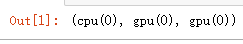x = nd.array([1, 2, 3])
x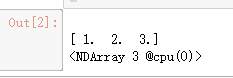x.context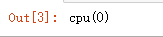a = nd.array([1, 2, 3], ctx=mx.gpu())
aB = nd.random.uniform(shape=(2, 3), ctx=mx.gpu(0))
B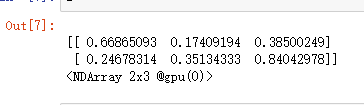y = x.copyto(mx.gpu())
yz = x.as_in_context(mx.gpu())
zy.as_in_context(mx.gpu()) is y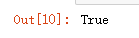copyto函数总是为目标变量开新的内存或显存。

y.copyto(mx.gpu()) is y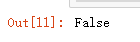MXNet的计算会在数据的context属性所指定的设备上执行。为了使用GPU计算，我们只需要事先将数据存储在显存上。计算结果会自动保存在同一块显卡的显存上。MXNet要求计算的所有输入数据都在内存或同一块显卡的显存上。

(z + 2).exp() * yGluon的模型可以在初始化时通过ctx参数指定设备。

net = nn.Sequential()
net(y)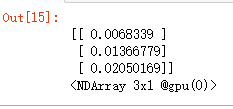net.weight.data()